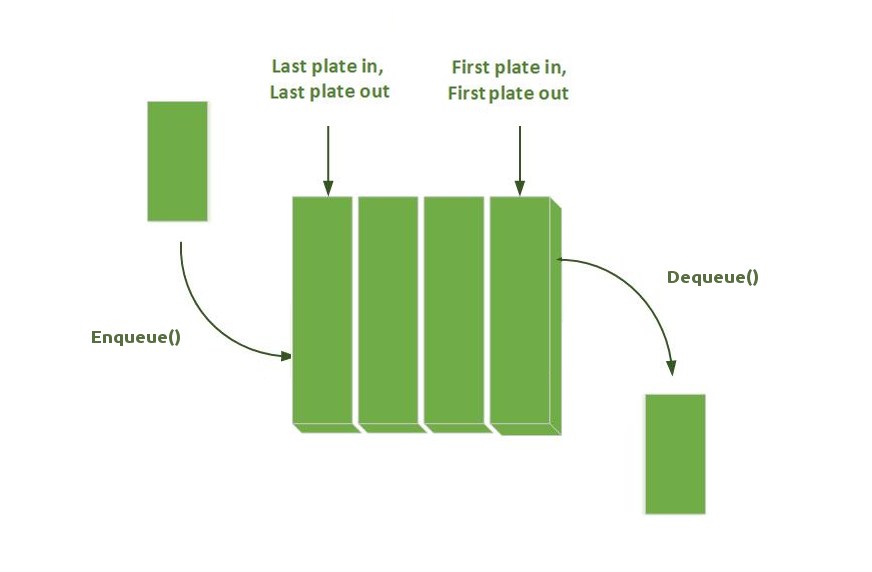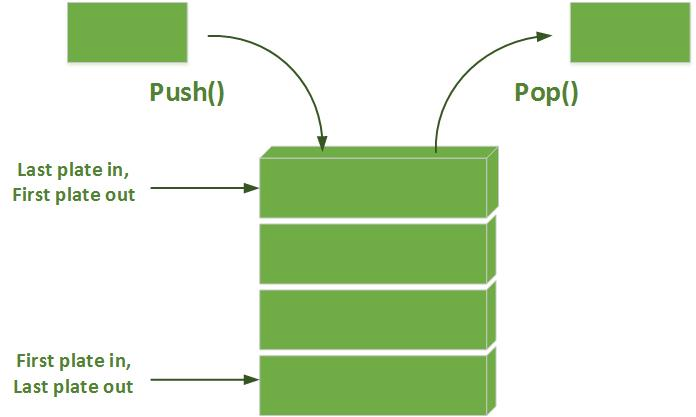Breadth First Search (BFS) is one of the two most fundamental graph traversal algorithms. First published in 1959 by Edward F. Moore to find the shortest path out of a maze, it is now used in a daily basis not only for regular traversal, but also for networks analysis, GPS, Search Engines, scheduling and other types of graph analysis.

In short : this is an incredibly useful algorithm that guarantees the shortest path between two nodes (when connections have the same cost).

Breadth First Search explores equally in all directions until the goal is reached. In other words, it starts from a chosen node and examine all its neighbors, then it examines all the neighbors of the neighbors, and so on... If we consider a tree (which is a simplified graph), the BFS will proceed as follows:

## Steps

• Add the start node in the queue and mark as visited.
• While there is a node waiting in the queue:
• 1. Take the node at the front of the line (queue).
• 2. Add to the queue all available neighbors, note the parent and mark as visited
• Done: backtrack from goal to start using parent link in order to get the shortest path.
BFS uses the opposite strategy as depth-first search (DFS), which instead explores the node branch as far as possible before being forced to backtrack. Still, both algorithms have the same lines of code; the only difference is the data structure used:

 BFS use a Queue (FIFO) DFS use a Stack (LIFO)This algorithm is, compared to the DFS, a little more tricky to implement in a recursive manner. As such we will only see the iterative approach ;)

## Code

BFS(Maze maze, Node start, Node end)
{
// Put the start node in the queue
start.visite = true;
Queue queue(start);

// While there is node to be handled in the queue
while (!queue.empty())
{
// Handle the node in the front of the lineand get unvisited neighbors
Node curNode = queue.pop();

// Terminate if the goal is reached
if (curNode == end)
break;

// Take neighbors, set its parent, mark as visited, and add to the queue
auto neighbors = curNode.GetUnvisitedNeighbors();
for (auto i = 0; i < neighbors.size(); ++i)
{
neighbors[i].visite = true;
neighbors[i].parent = curNode;
queue.push(neighbors[i]);
}
}

// Done ! At this point we just have to walk back from the end using the parent
// If end does not have a parent, it means that it has not been found.
}